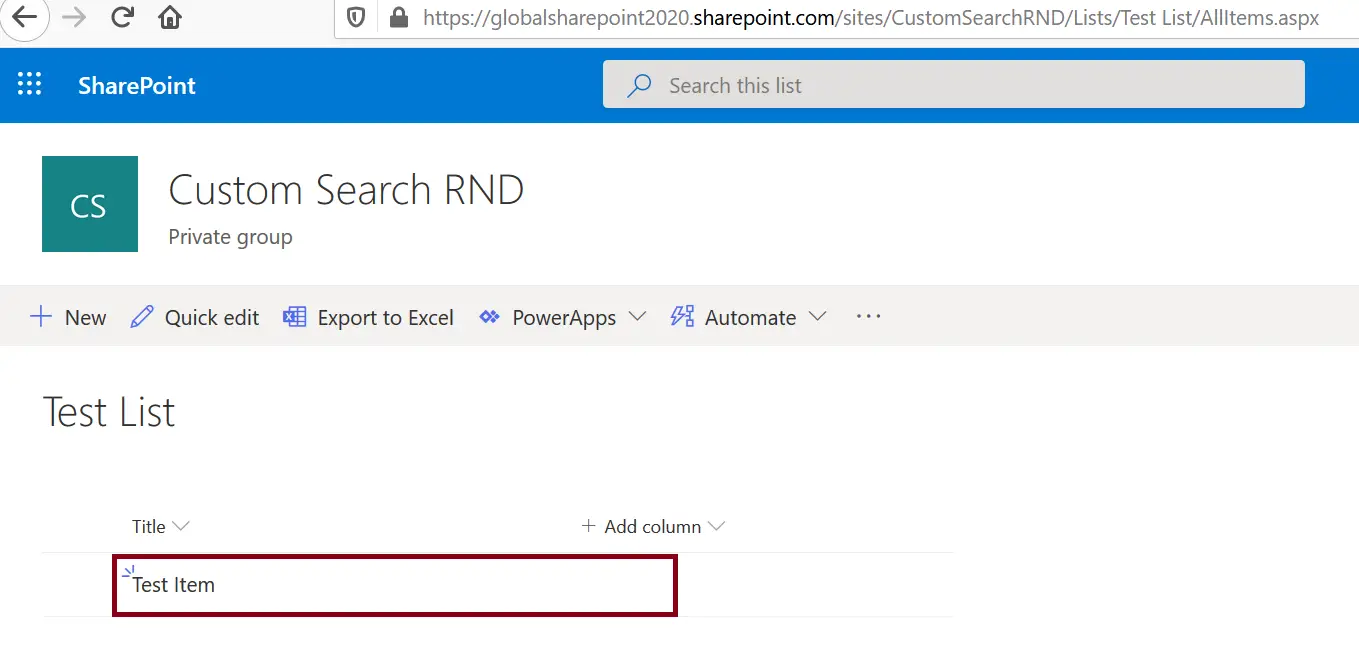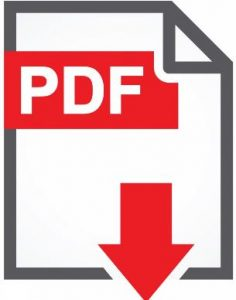# [Proven Top 25 plus]: Validate special characters in SharePoint list column(special character validation in SharePoint)

42,713 total views,  5 views today

Data Validation in Excel - Create a...
Data Validation in Excel - Create a Drop Down menu in Excel

Top 25 plus special characters in SharePoint – sometimes, we need to validate the special characters in SharePoint list columns – so that we can prevent the user to enter special characters in the SharePoint column while entering the data. Here in this tutorial, we will learn about how to validate special characters in the SharePoint list column(special character validation in SharePoint).

## Validate special characters in SharePoint online

It is very simple to validate with just a few clicks and with the validation formula.

Step 1:

Go to the List Settings page, then click on the column for which you want to add the validation formula. For example, here I have selected the column “Title“.

Step 2:

Click on the column name for which you want to add the validation formula – here I have clicked on the “Title” column which will take users to the “Edit Column” page.

Go to the “Column Validation” section and in the “Formula” box add the below formula and in the “User message” box, you can give your validation message which is optional but better to provide.

Syntax:

```=AND(IF(ISERROR(FIND(",",[ColumnName])),TRUE),
IF(ISERROR(FIND("&",[ColumnName])),TRUE),
IF(ISERROR(FIND(";",[ColumnName])),TRUE),
IF(ISERROR(FIND("[",[ColumnName])),TRUE),
IF(ISERROR(FIND("+",[ColumnName])),TRUE),
IF(ISERROR(FIND(":",[ColumnName])),TRUE),
IF(ISERROR(FIND(")",[ColumnName])),TRUE),
IF(ISERROR(FIND("-",[ColumnName])),TRUE),
IF(ISERROR(FIND("*",[ColumnName])),TRUE),
IF(ISERROR(FIND("(",[ColumnName])),TRUE),
IF(ISERROR(FIND("\$",[ColumnName])),TRUE),
IF(ISERROR(FIND("%",[ColumnName])),TRUE),
IF(ISERROR(FIND("~",[ColumnName])),TRUE),
IF(ISERROR(FIND("#",[ColumnName])),TRUE),
IF(ISERROR(FIND("]",[ColumnName])),TRUE),
IF(ISERROR(FIND(".",[ColumnName])),TRUE),
IF(ISERROR(FIND("!",[ColumnName])),TRUE),
IF(ISERROR(FIND("@",[ColumnName])),TRUE),
IF(ISERROR(FIND("/",[ColumnName])),TRUE),
IF(ISERROR(FIND("\",[ColumnName])),TRUE))```

Notes:

• In the above formula, replace “ColumnName” with your column name.
• You can add or remove validation from the above formula based on your requirement.
• For the addition of a new validation condition, you can add a special character like the below and include this line to the above formula.
`IF(ISERROR(FIND("speacial character you want to add",[ColumnName])),TRUE),`
• For the deletion of the existing validation condition, just we need to remove the “IF” condition line from the above-consolidated formula.

Example:

I have applied the validation formula in the “Title” column.

```=AND(IF(ISERROR(FIND(",",[Title])),TRUE),
IF(ISERROR(FIND("&",[Title])),TRUE),
IF(ISERROR(FIND(";",[Title])),TRUE),
IF(ISERROR(FIND("[",[Title])),TRUE),
IF(ISERROR(FIND("+",[Title])),TRUE),
IF(ISERROR(FIND(":",[Title])),TRUE),
IF(ISERROR(FIND(")",[Title])),TRUE),
IF(ISERROR(FIND("-",[Title])),TRUE),
IF(ISERROR(FIND("*",[Title])),TRUE),
IF(ISERROR(FIND("(",[Title])),TRUE),
IF(ISERROR(FIND("\$",[Title])),TRUE),
IF(ISERROR(FIND("%",[Title])),TRUE),
IF(ISERROR(FIND("~",[Title])),TRUE),
IF(ISERROR(FIND("#",[Title])),TRUE),
IF(ISERROR(FIND("]",[Title])),TRUE),
IF(ISERROR(FIND(".",[Title])),TRUE),
IF(ISERROR(FIND("!",[Title])),TRUE),
IF(ISERROR(FIND("@",[Title])),TRUE),
IF(ISERROR(FIND("/",[Title])),TRUE),
IF(ISERROR(FIND("\",[Title])),TRUE))```

## Test – Demo: validate special characters in SharePoint online (character limit in SharePoint list)

After adding the validation formula to the “Title” column when we try to enter an item with a special character in the “Title” column, we will not be able to save the data and the user will get the validation message “Please enter the valid data”.

In the above example, we have entered the “Test . Item” where dot “.”  is the special character as per the above validation formula which we have applied, so it does not allow to save the data, however, once we remove the dot “.” from the “Title” column value, it will be saved successfully, please see the below:Column validation in SharePoint Online list – test successful

## Summary: validate special characters in SharePoint online (SharePoint special characters)

Thus, in this blog, we have learned about how to validate the special characters in the SharePoint list column and prevent being entered into the list item.